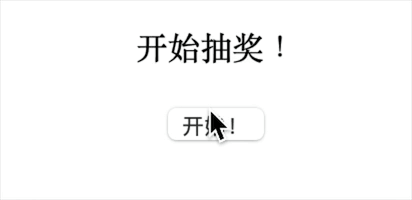# 涉及到的技术栈/框架

• 前端开发基础知识（Html/Css/JavaScript）
• Javascript ES6语法
• Vue.js相关技术知识
• JavaScript异步知识

# 基础代码

``````<!DOCTYPE html>
<html lang="zh">

<meta charset="UTF-8">
<meta http-equiv="X-UA-Compatible" content="IE=edge">
<meta name="viewport" content="width=device-width, initial-scale=1.0">
<title>Lottery</title>
<style>
#app {
margin: auto;
width: 200px;
text-align: center;
}
</style>

<body>
<div id="app">
<p style="height:22px">{{item}}</p>
<button @click="start">开始！</button>
</div>

<script src="//cdn.jsdelivr.net/npm/[email protected]/dist/vue.js"></script>
</body>
<script>
const app = new Vue({
el: "#app",
data: {
list: ['1积分', '5积分', '10积分', '20积分', '谢谢惠顾', '再来一次'],
item: "开始抽奖！"
},
methods: {
start() {
for (let index = 0; index < 50; index++) {
setTimeout(() => {
this.item = this.list[Math.round(Math.random() * (this.list.length - 1))]
}, 100 + index * 100)

}

}
}
})
</script>

</html>
``````

data中，list数组 作为奖池，用于放置可以被抽取到的item，item变量 则用来指明当前抽取到的item并与用于显示当前item的 段落元素 进行绑定 ，在 start方法 执行之前，item变量 默认值为“开始抽奖！”。

methods中只有一个 start方法 用于启动抽奖。通过 for循环 ，进行50次的抽取。setTimeOut 将抽取时间控制在100毫秒，也就是说每100毫秒进行一次抽取，从 list数组 中随机抽取值替换当前的 item变量# 需求

1. 优化抽取过程，使抽取过程流畅不那么僵硬
2. 优化抽取规则，使抽取规则更为严谨
3. 优化完善部分细节

# 完善过程

## 优化抽取过程

• start 方法的更新：
``````            async start() {
for (let index = 0; index < 50; index++) {
this.item = await new Promise(resolve => {
setTimeout(() => {
resolve(this.list[Math.round(Math.random() * (this.list.length - 1))])
}, Math.round(this.getTime(index)))
})
}
},
``````

• 新增 getTime 方法
``````            getTime(index) {
// 初始/正常间隔时间
let time = 80
// 启动间隔时间处理
if (index <= 10) {
time = time + (11 - index) * 20
}
// 结束间隔时间的处理
if (index >= 39) {
time = time + (index - 39) * 20
if (index === 49) time = 1000
}
return time
}
``````

## 优化抽取规则

• start 方法的更新：
``````            async start() {
for (let index = 0; index < 50; index++) {
this.item = await new Promise(resolve => {
setTimeout(() => {
resolve(this.getItem())
}, Math.round(this.getTime(index)))
})
}
},
``````

• 新增 getItem 方法
``````            getItem() {
let newItem;
do {
newItem = this.list[Math.round(Math.random() * (this.list.length - 1))]
} while (this.item === newItem);
return newItem
}
``````

## 优化细节

``````        data: {
startButtonDisabled: false,
finished: false,
list: ['1积分', '5积分', '10积分', '20积分', '谢谢惠顾', '再来一次'],
item: "开始抽奖！"
},
``````

data 中加入 startButtonDisabledfinished 用来分别记录按钮状态以及开奖的状态。

``````            handleStart(index) {
if (index === 0) {
this.finished = false
this.startButtonDisabled = true
}
},
handleEnd(index) {
if (index === 49) {
this.finished = true
this.startButtonDisabled = false
}
}
``````

``````            async start() {
for (let index = 0; index < 50; index++) {
this.handleStart(index)
this.item = await new Promise(resolve => {
setTimeout(() => {
resolve(this.getItem())
}, Math.round(this.getTime(index)))
})
this.handleEnd(index)
}
},
``````

start 方法的循环体首尾调用 handleStarthandleEnd 方法。

``````  <div id="app">
<p :class="{active:finished}" style="height:22px">{{item}}</p>
<button :disabled="startButtonDisabled" @click="start">开始！</button>
</div>
``````

``````        p.active {
font-weight: bold;
color: red
}
``````

# 最终代码

``````<!DOCTYPE html>
<html lang="zh">

<meta charset="UTF-8">
<meta http-equiv="X-UA-Compatible" content="IE=edge">
<meta name="viewport" content="width=device-width, initial-scale=1.0">
<title>Lottery</title>
<style>
#app {
margin: auto;
width: 200px;
text-align: center;
}

p.active {
font-weight: bold;
color: red
}
</style>

<body>
<div id="app">
<p :class="{active:finished}" style="height:22px">{{item}}</p>
<button :disabled="startButtonDisabled" @click="start">开始！</button>
</div>
<script src="//cdn.jsdelivr.net/npm/[email protected]/dist/vue.js"></script>
</body>
<script>
const app = new Vue({
el: "#app",
data: {
startButtonDisabled: false,
finished: false,
list: ['1积分', '5积分', '10积分', '20积分', '谢谢惠顾', '再来一次'],
item: "开始抽奖！"
},
methods: {
async start() {
for (let index = 0; index < 50; index++) {
this.handleStart(index)
this.item = await new Promise(resolve => {
setTimeout(() => {
resolve(this.getItem())
}, Math.round(this.getTime(index)))
})
this.handleEnd(index)
}
},
getTime(index) {
// 初始/正常间隔时间
let time = 80
// 启动间隔时间处理
if (index <= 10) {
time = time + (11 - index) * 20
}
// 结束间隔时间的处理
if (index >= 39) {
time = time + (index - 39) * 20
if (index === 49) time = 1000
}
return time
},
getItem() {
let newItem;
do {
newItem = this.list[Math.round(Math.random() * (this.list.length - 1))]
} while (this.item === newItem);
return newItem
},
handleStart(index) {
if (index === 0) {
this.finished = false
this.startButtonDisabled = true
}
},
handleEnd(index) {
if (index === 49) {
this.finished = true
this.startButtonDisabled = false
}
}
}
})
</script>

</html>
``````